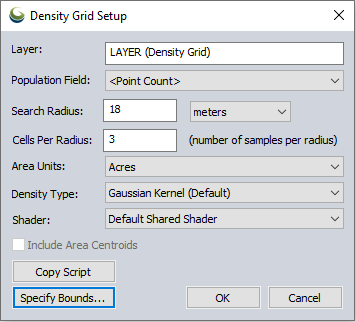#Create Density Grid (Heat Map) from Point Data

This tool create a new layer by calculating density values from the point features in the selected layer. You can setup the density grid to be based on a simple point count or as a sum of the numeric values of some attribute value for the points within some search radius. The Density Grid Setup dialog (pictured below) appears when you select this option, allowing you to control the density grid calculation.Population Field - This option allows you to control whether a simple point count or attribute value is used to compute the density grid.

Search Radius - Used to specify how far from a grid sample location a point can be and still be considered as part of the value.

Cells per Radius - When combined with the Search Radius, controls how large the pixels are in the resulting density grid. For example, if you have a search radius of 90 meters and a 'Cells Per Radius' of 3, each pixel should end up 30 meters across.

Density Type This option allows you to specify whether a weighted-distance Gaussian distribution is used (this gives much prettier results) or a simple in-or-out of radius calculation is used.

Shader - Use this option to control which elevation shader is used to render the computed grid. You will often want to select